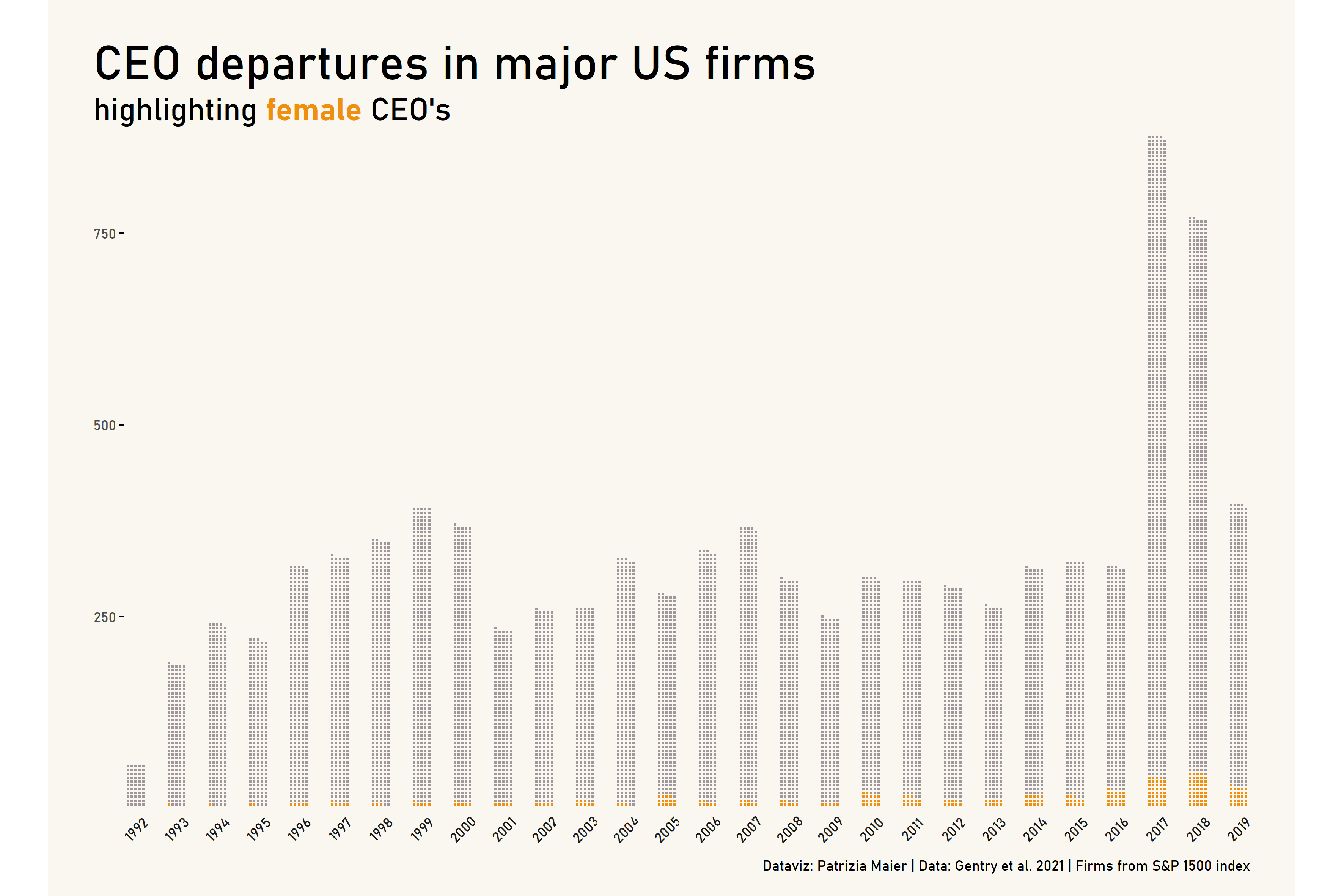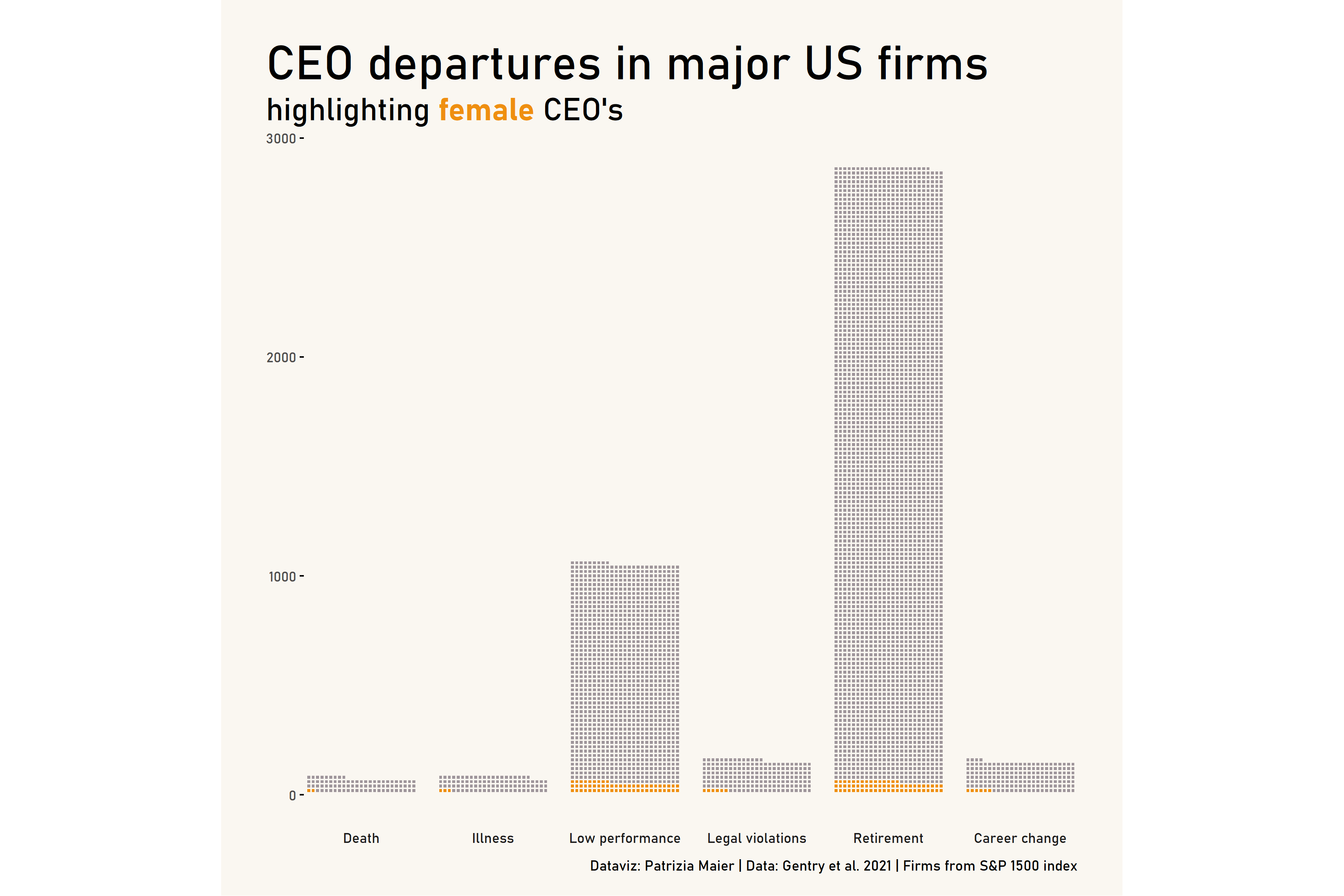# Plot No. 1

By Patrizia Maier

``````# get packages
library(tidyverse)
library(gender)
library(genderdata)
library(extrafont)
library(ggtext)
library(waffle)
# font_import() # only once
loadfonts(device = "win", quiet = TRUE) # every time
# windowsFonts() # to check available options
``````
``````# get tidy tuesday data
departures <- tuesdata\$departures
``````
``````# text mining gender information (only male/female)
# extract names
names <- departures\$exec_fullname %>%
str_split_fixed(pattern=" ", n=5) %>% # split names into 5 column matrix
as_tibble() %>% # convert to tibble
mutate(first_name=case_when(
str_detect(V1, "\\.") ~ V2, # if abbreviated first name, get second name
TRUE ~ V1 # else first name))
``````
``````# perform gender guess prediction
gender_guess <- names\$first_name %>%
gender(years = c(1920, 1985), method = "ssa") %>% # apply gender estimation
select(!starts_with("year")) %>% # select variables
distinct() %>%  # remove duplicates
rename("gender_name"="gender") # rename variable for clarity
``````
``````# Caution: Some names are ambiguous and can be female or male (see proportion).
# In the data set, quite a few CEO's with highly likely "female" names are actually "male".
# This is implied by the information in 'notes' (e.g., "Mr. ", "he", ...).
# Besides, there are quite a few NA's in case of abbreviated first names (e.g., "L.").
# --> Therefore we need more text mining based on 'notes'.
``````
``````# add information from 'notes'
indicator_male <- c("\\Whe\\W", "\\Whe's\\W","\\Whis\\W", "\\WMr\\W")
indicator_female <- c("\\Wshe\\W", "\\Wshe's\\W", "\\Wher\\W", "\\WMrs\\W", "\\WMs\\W", "\\Wlady\\W")
``````
``````departures <- departures %>%
bind_cols(names) %>%
left_join(gender_guess, by=c("first_name"="name")) %>%
mutate(gender_notes_fem=str_detect(notes, regex(paste(indicator_female, collapse = "|"), ignore_case = T)),
gender_notes_male=str_detect(notes, regex(paste(indicator_male, collapse = "|"), ignore_case = T))) %>%
mutate(final_gender_guess=case_when(
proportion_male > 0.99 ~ "male",
proportion_female > 0.99 ~ "female",
gender_notes_male & !gender_notes_fem ~ "male",
gender_notes_fem & !gender_notes_male ~ "female",
!gender_notes_male & !gender_notes_fem ~ gender_name)) %>%
mutate(final_gender_guess=as.factor(final_gender_guess))
``````
``````# summary(departures\$final_gender_guess)
# female   male   NA's
#   299   8850    274
``````
``````# prepare data for plotting
data_gender <- departures %>%
group_by(fyear) %>%
count(final_gender_guess) %>%
drop_na(final_gender_guess) %>%
complete(final_gender_guess, fill=list(n=0)) %>%
filter(fyear>=1992 & fyear <2020)
``````
``````# create waffle plot
p1 <- ggplot(data_gender, aes(fill = final_gender_guess, values = n)) +
geom_waffle(color = "#faf7f1", size = .5, n_rows = 5, flip = TRUE) +
facet_wrap(~fyear,  nrow = 1, strip.position = "bottom") +
scale_fill_manual(values = c("#ef8f10", "#9f969b")) +
scale_x_discrete() +
scale_y_continuous(labels = function(x) x * 5, # make this multiplier the same as n_rows
expand = c(0,0)) +
coord_equal(clip="off") +
theme_minimal() +
theme(plot.background = element_rect(fill = "#faf7f1", linetype = "blank"),
plot.title = element_markdown(size=30),
plot.title.position = "plot",
plot.subtitle = element_markdown(size=20),
plot.caption = element_markdown(size=9),
plot.caption.position = "plot",
plot.margin = unit(c(1,1,0.5,1), "cm"),
panel.grid = element_blank(),
panel.spacing.x = unit(0.75, "lines"),
axis.ticks.y = element_line(),
legend.position = "none",
legend.title = element_blank(),
legend.direction = "horizontal",
text=element_text(family = "Bahnschrift", size=11),Bonus Plot: